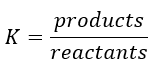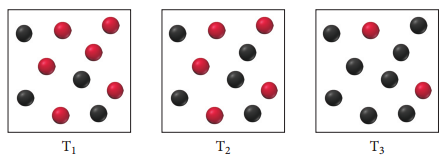# Problem: Consider the reaction A(g) ⇌ B(g). The images shown here illustrate equilibrium mixtures of A (red) and g (black) at three different temperatures.At which temperature is the equilibrium constant the largest?

###### FREE Expert Solution

Recall the expression for the equilibrium constant K:Let’s estimate the value of the equilibrium constant in each temperature:

Reactant = red spheres

Product = black spheres

100% (343 ratings)###### Problem Details
Consider the reaction A(g) ⇌ B(g). The images shown here illustrate equilibrium mixtures of A (red) and g (black) at three different temperatures.At which temperature is the equilibrium constant the largest?